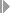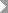Show TOC

###Calculation of Target Costs#### Use

The system calculates target costs so that the same basis is used to determine the variances between the control costs and the planned costs. The planned costs are adjusted to the actual costs by dividing the confirmed quantity by the planned quantity.

#### Prerequisites

The calculation of target costs is controlled by a target cost version that you define in Customizing for Product Cost by Period or Product Cost by Order .

#### Features

How the target costs are calculated depends on the target cost version:

• Target cost version 0 (variances relevant to settlement)

Costs in the standard cost estimate for the material that vary with the lot size are divided by the costing lot size and multiplied by the quantity delivered (the yield).

Costs that do not vary with the lot size (such as setup costs) are used directly as target costs.

• Target cost version 1 (production variances)

Costs in the order plan that vary with the lot size are divided by the planned order lot size and multiplied by the quantity delivered (the yield).

Costs that do not vary with the lot size (such as setup costs) are used directly as target costs.

• Target cost version 2 (planning variances)

Costs in the standard cost estimate for the material that vary with the lot size are divided by the costing lot size and multiplied by the order plan quantity.

Costs that do not vary with the lot size (such as setup costs) are used directly as target costs.

• Target cost version 3 (production variances against planned price of period)

Costs that vary with the lot size are divided by the costing lot size of the modified standard cost estimate and multiplied by the quantity delivered (the yield) of the period.

Costs that do not vary with the lot size (such as setup costs) are used directly as target costs.

Base Quantity

In target cost versions 0, 1, and 3, the target costs are calculated on the basis of the quantity delivered (the yield) per order. This has the following consequences:

• In cumulative variance calculation, target costs can only be calculated for production orders that have the status DLV ( Delivered ) or TECO ( Technically completed ).

• In periodic variance calculation, target costs can only be calculated for production orders for which a goods receipt was posted in that period.

In target cost version 2 the target costs are calculated using the planned order quantity.

Base Costs

In make-to-stock production, target cost versions 0 and 2 calculate the target costs using the standard cost estimate for the material being produced.

In sales-order-related production with a valuated sales order inventory, target cost versions 0 and 2 calculate the target costs using the strategy sequence on which the valuation of the valuated sales order inventory is based. The system first attempts to select a sales order cost estimate.

The costs calculated in this way are used to calculate the standard price. Only the costs flagged in the cost component view as relevant for inventory valuation are included in this price.

The standard cost estimate can also contain sales and distribution costs that are not relevant for inventory valuation. In costing, these costs are shown in a separate cost component view. Such costs are not used for target cost calculation on the basis of the standard cost estimate. This ensures that the target costs for the withdrawal of a semifinished product are the same as the actual costs.

Breakdown into Fixed and Variable Costs

In target cost calculation, the costs can be broken down into fixed and variable costs. A requirement for this function is that the price control indicator in the material’s master record is set to S (standard price) and that a current standard cost estimate for the material exists.

Inclusion of Costs Relevant to Inventory Valuation

The standard cost estimate for the material and the sales order cost estimate can also contain sales and distribution costs that are not relevant for inventory valuation. These costs are shown in a separate cost component view. Such costs are not included when the target costs are calculated. This ensures that the target costs for the withdrawal of a semifinished product are the same as the actual costs.

You can use the calculation base in the costing type for the standard cost estimate to choose a cost component view that is used for the calculation of the material overhead view. For variance calculation, you should make sure that calculation base 06 is set for inventory valuation.

For information on target cost versions, refer to the IMG for Product Cost by Period and Product Cost by Order underPeriod-End ClosingVariances.